# Angular ngFor Directive

## Angular ngFor Directive with Examples

In this article, I am going to discuss the Angular ngFor Directive with an example. Please read our previous article where we discussed the basics of Angular Directives. The Angular Directives are one of the most important features of Angular applications. They are used to extend the power of HTML attributes. At the end of this article, you will understand what exactly angular ngFor directive is and when and how to use this directive in Angular Application.

##### What is Angular ngFor Directive?

The built-in ngFor directive belongs to the Structural directive category. As it belongs to the structural directive category, it is used to change the structure of the DOM.

The ngFor directive is very much similar to the “for loop” used in most of the programming languages. So, the NgFor directive is used to iterate over a collection of data. The syntax to use ngFor directive is: *ngFor=”let <value> of <collection>”

Here, <value> is a variable name and collection is a property on your component which a collection of data. Usually an array but it can be anything that can be iterated over in a for loop.

##### Understanding Angular ngFor Directive:

Let us understand the ngFor structural directive in angular application with an example. We are going to use the following array of Student objects in this demo.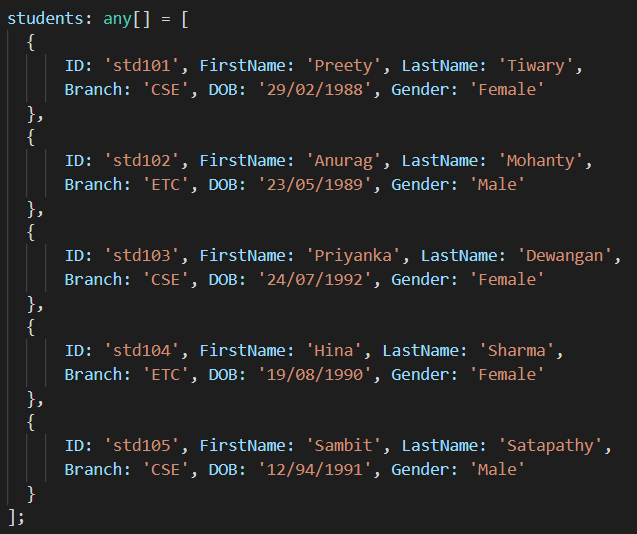Then we want to display the above students in a table on a web page as shown below.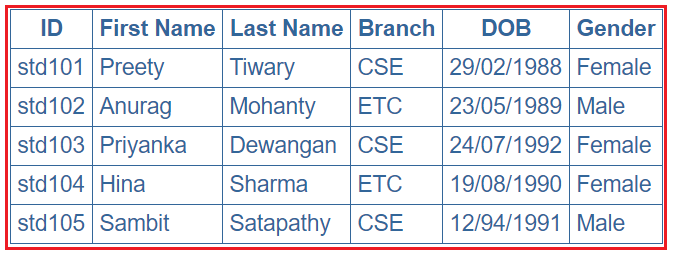Let’s discuss the Step By Step Procedure to achieve the above output using Angular ngFor Directive:

##### Step1: Modify the app.component.ts file

Open app.component.ts file and then copy and paste the following code in it. You can find this file within the app folder of your angular project.

```import { Component } from '@angular/core';
@Component({
selector: 'app-root',
templateUrl: './app.component.html',
styleUrls: ['./app.component.css']
})
export class AppComponent {

students: any[] = [
{
ID: 'std101', FirstName: 'Preety', LastName: 'Tiwary',
Branch: 'CSE', DOB: '29/02/1988', Gender: 'Female'
},
{
ID: 'std102', FirstName: 'Anurag', LastName: 'Mohanty',
Branch: 'ETC', DOB: '23/05/1989', Gender: 'Male'
},
{
ID: 'std103', FirstName: 'Priyanka', LastName: 'Dewangan',
Branch: 'CSE', DOB: '24/07/1992', Gender: 'Female'
},
{
ID: 'std104', FirstName: 'Hina', LastName: 'Sharma',
Branch: 'ETC', DOB: '19/08/1990', Gender: 'Female'
},
{
ID: 'std105', FirstName: 'Sambit', LastName: 'Satapathy',
Branch: 'CSE', DOB: '12/94/1991', Gender: 'Male'
}
];
}
```

As you can see in the above code, we have created one array (i.e. students) which a collection of student data. Again we have specified the templateUrl and StyleUrls, so, let proceed and modifies these two files.

##### Step2: Modify app.component.css file

Open app.component.css file and then copy and paste the following code in it. You can find this file within your app folder. The following styles are going to be used in our HTML page to style table data.

```table {
color: #369;
font-family: Arial, Helvetica, sans-serif;
font-size: large;
border-collapse: collapse;
}

td {
border: 1px solid #369;
}

th{
border: 1px solid #369;
}
```
##### Step3: Modify app.component.html file

Open app.component.html file and then copy and paste the following code in it. You can also find this file within your app folder.

```<table>
<tr>
<th>ID</th>
<th>First Name</th>
<th>Last Name</th>
<th>Branch</th>
<th>DOB</th>
<th>Gender</th>
</tr>
<tbody>
<tr *ngFor='let student of students'>
<td>{{student.ID}}</td>
<td>{{student.FirstName}}</td>
<td>{{student.LastName}}</td>
<td>{{student.Branch}}</td>
<td>{{student.DOB}}</td>
<td>{{student.Gender}}</td>
</tr>
<tr *ngIf="!students || students.length==0">
<td colspan="7">
No Students to display
</td>
</tr>
</tbody>
</table>
```
##### Understand the above Code:
1. Here, the ngFor directive is used to iterate over a collection. In this example, the collection is an array of students.
2. As the ngFor directive is a structural directive, so it is prefixed with * (star). So, the point that you need to remember is, all the structural directive are prefixed with a *.
3. *ngFor=’let student of students’ – In this statement, the ‘student‘ is called template input variable, which can be accessed by the <tr> element and any of its child elements.
4. The ngIf structural directive displays the row “No Students to display” when the student’s property does not exist or when there are no students in the array. We will discuss this directive in detail in our upcoming articles.
##### Step4: Modify app.module.ts file

Open app.module.ts file which is present inside the app folder and then copy and paste the following code.

```import { BrowserModule } from '@angular/platform-browser';
import { NgModule } from '@angular/core';
import { AppRoutingModule } from './app-routing.module';
import { AppComponent } from './app.component';
import { FormsModule } from '@angular/forms';

@NgModule({
declarations: [
AppComponent
],

imports: [
BrowserModule,
AppRoutingModule,
FormsModule
],

providers: [],
bootstrap: [AppComponent]
})

export class AppModule { }
```

That’s it. We have done with our implementation. Now run the application and you will notice that the students are displayed in the table as expected.

##### ngFor – Local Variables:

The ngFor structural directive has the following local variable which you can use to customize the data.

1. Index: This variable is used to provide the index position of the current element while iteration.
2. First: It returns boolean true if the current element is the first element in the iteration else it will return false.
3. Last: It returns boolean true if the current element is the last element in the iteration else it will return false.
4. Even: It returns boolean true if the current element is even element based on the index position in the iteration else it will return false.
5. Odd: It returns boolean true if the current element is an odd element based on the index position in the iteration else it will return false.

Let us understand the above ngFor local variables one by one with example.

##### How to get the index of an item in a collection in an angular application?

We can get the index of an item in a collection using the index property of the ngFor directive. Let us understand this with an example. What we want to do here is, along with the student data, we also want to display the index position as shown in the below image.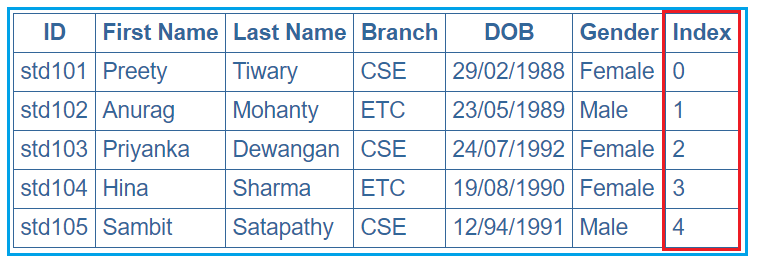In order to achieve this, we want to use the ngFor local variable index. So, modify the app.component.html as shown below.

```<table>
<tr>
<th>ID</th>
<th>First Name</th>
<th>Last Name</th>
<th>Branch</th>
<th>DOB</th>
<th>Gender</th>
<th>Index</th>
</tr>
<tbody>
<tr *ngFor='let student of students; let i=index'>
<td>{{student.ID}}</td>
<td>{{student.FirstName}}</td>
<td>{{student.LastName}}</td>
<td>{{student.Branch}}</td>
<td>{{student.DOB}}</td>
<td>{{student.Gender}}</td>
<td>{{i}}</td>
</tr>
</tbody>
</table>
```

Notice that in the above code, we are using the index property of the Angular ngFor directive to store the index position of an element in a template input variable “i”. The variable “i” is then used in the <td> element where we want to display the index value. We used the let keyword to create the template input variable “i”.  The let keyword in angular is very much similar to the var keyword.

The index of an element is extremely useful when you are creating the HTML elements dynamically. We will discuss creating the HTML elements dynamically in our upcoming articles.

##### How to identify the first and the last elements in a collection in an angular application?

To identify the First and Last elements in a collection, you need to use the first and last properties of the ngFor directive respectively. Let us understand this with an example. We want to display the student data along with whether that student is the first or the last student as shown in the below image.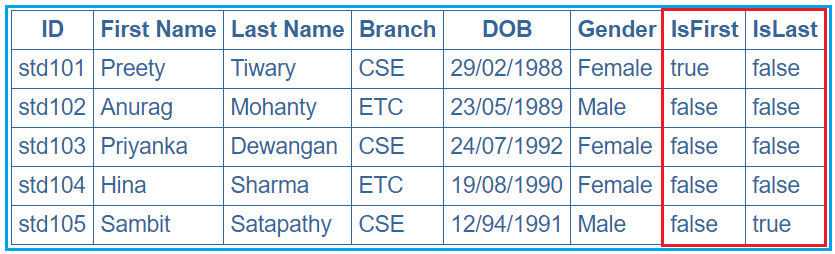In order to achieve this, modify the app.component.html file as shown below.

```<table>
<tr>
<th>ID</th>
<th>First Name</th>
<th>Last Name</th>
<th>Branch</th>
<th>DOB</th>
<th>Gender</th>
<th>IsFirst</th>
<th>IsLast</th>
</tr>
<tbody>
<tr *ngFor='let student of students; let isFirst = first; let isLast = last'>
<td>{{student.ID}}</td>
<td>{{student.FirstName}}</td>
<td>{{student.LastName}}</td>
<td>{{student.Branch}}</td>
<td>{{student.DOB}}</td>
<td>{{student.Gender}}</td>
<td>{{isFirst}}</td>
<td>{{isLast}}</td>
</tr>
</tbody>
</table>
```
##### How to identify the even and odd elements in a collection in an angular application?

In order to identify the Event and Odd elements in a collection in angular, you need to use the even and odd local variable of the ngFor directive respectively. Let us understand this with an example. We want to display the student data along with whether that student is odd or even as shown in the below image.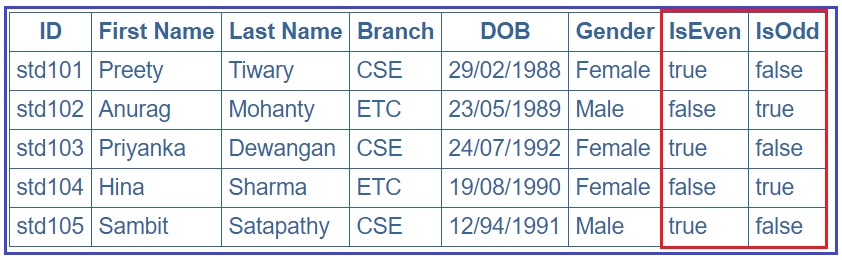In order to achieve this, modify the app.component.html file as shown below.

```<table>
<tr>
<th>ID</th>
<th>First Name</th>
<th>Last Name</th>
<th>Branch</th>
<th>DOB</th>
<th>Gender</th>
<th>IsEven</th>
<th>IsOdd</th>
</tr>
<tbody>
<tr *ngFor='let student of students; let isEven = even; let isOdd = odd'>
<td>{{student.ID}}</td>
<td>{{student.FirstName}}</td>
<td>{{student.LastName}}</td>
<td>{{student.Branch}}</td>
<td>{{student.DOB}}</td>
<td>{{student.Gender}}</td>
<td>{{isEven}}</td>
<td>{{isOdd}}</td>
</tr>
</tbody>
</table>
```

1.2.